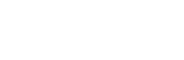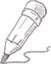#CDA持证人阿涛

2022-12-30   阅读量: 223

Mysql

## mysql 学习52--产品贡献定量分析（帕累托分析）-- 3.3 产品贡献定量分析（帕累托分析）

##累积销售额占比=累积销售额/总销售额

select *

from

(select

item_category,

sum(amount) as 销售额,

sum(sum(amount)) over(order by sum(amount) desc) as 累积销售额,

#当over中指定了排序,但是没有指定分区和指定滑动窗口范围时,默认计算当前分区内第一行到当前行(排序字段)取值范围内的记录

sum(sum(amount)) over() as 总销售额,

#当over中没有指定分区、排序和滑动窗口范围时,表中所有记录为一个区,默认计算当前分区内的所有记录

sum(sum(amount)) over(order by sum(amount) desc)/sum(sum(amount)) over() as 累积销售额占比

from userbehavior_new

group by item_category) as t

where 累积销售额占比<=0.8;

34.2857 2 0 关注作者 收藏

## 评论(0)